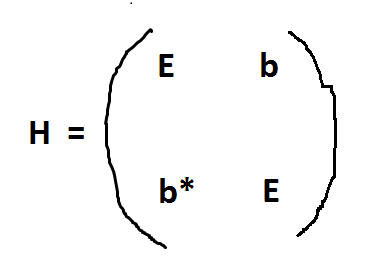# Perturbation Theory: Calculating 1st-Order Correction

• ooleonardoo

#### ooleonardoo

Homework Statement
Calculate the second-order corrections to energy for the following Hamiltonian matrix.
Use the degenerate perturbation theory. Consider 'b' as perturbation.
Relevant Equations
...
Of course, this question consisted of two parts. In the first part, we needed to calculate the first-order correction. It was easy. In all the books on quantum mechanics I saw, only first-order examples have been solved. So I really do not know how to solve it. Please explain the solution method to me. ThankfulWhat are those books you mention? I would say Sakurai and probably a lot of other books do actually explain how to do PT to arbitrary orders.

What are those books you mention? I would say Sakurai and probably a lot of other books do actually explain how to do PT to arbitrary orders.
gasiorowicz, zettili , griffiths
The level of the book you mentioned is high for me. Do you know of any other book that explains this with an example?

Actually, Griffiths does have a discussion on second-order PT.
Anyway, can you show us how did you compute the first-order correction?